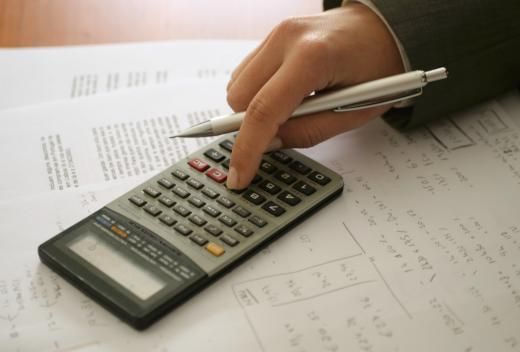Finance
Fact Checked

# What is an Effective Interest Rate?

A. Gabrenas
A. Gabrenas

Effective interest rate, also called an annual effective rate or AER, refers to the actual amount of interest paid when taking compounding periods into effect. It often varies from annual percentage rate, which is the interest rate usually stated in credit terms, because APR generally does not account for compounding periods. Calculating the effective interest rate can often give a person a more accurate idea of the amount of interest he or she will pay over the life of a loan or receive from an investment.

To determine the effective interest rate on a set of credit terms, a key value to have is how often the interest compounds. Then, the nominal interest rate must be determined by diving the APR by the number of compounding periods. For example, if a credit card charges 15% APR and compounds interest every month, the nominal interest rate would be 1.25% a month. A person can then calculate the effective interest rate by using the formula: [(1+I)^C - 1] x 100, where I is equal to the nominal interest rate expressed in decimal form and C is equal to the number of compounding periods expressed in whole numbers. For the credit card terms previously referenced, this would be[(1 + 0.0125)^12 -1] x 100, or 16.07%.Calculating the effective interest rate can often give a person a more accurate idea of the amount of interest he or she will pay over the life of a loan or receive from an investment.

As the above example illustrates, the effective interest rate is often higher than the APR due to the compounding effects. When it comes to borrowing money, this generally means a person will pay more over the long run as the frequency of compounding increases. Conversely, when it comes to investing, it can mean a person makes more over the long run as compounding increases.

Being able to calculate the effective interest rate can be helpful when comparing similar credit offers. Offers may have the same APR, but drastically different compounding rates, which affects the overall payback in the case of a loan, or payout, in the case of an investment. For example, take two short-term payday loans offering \$1,000 U.S. dollars at 25% APR to be paid back over one year.

The first loan does not compound interest, which means the effective interest rate is also 25% and the borrower would owe \$1,250 USD at the end of a year. The second loan compounds interest once a month, raising the effective interest rate to about 28% and bringing the total owed to \$1,280. In this scenario, while both loans seem to have the same interest rate at the outset, calculating the effective rate clarifies the better loan terms.

## You might also Like